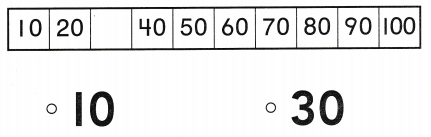# Texas Go Math Kindergarten Lesson 16.3 Answer Key Count to 100 by Tens

Refer to our Texas Go Math Kindergarten Answer Key Pdf to score good marks in the exams. Test yourself by practicing the problems from Texas Go Math Kindergarten Lesson 16.3 Answer Key Count to 100 by Tens.

## Texas Go Math Kindergarten Lesson 16.3 Answer Key Count to 100 by Tens

Essential Question
How can you count to 100 by tens on a hundred chart?

ExploreExplanation:
The given pattern is count by tens
10 , 20 , 30 , 40 , 50 , 60 , 70 , 80 , 90 , 100

Directions
Trace the circles around the numbers that end in a 0. Beginning with 10, count those numbers in order. Tell a friend how you are counting.

Share and Show

Question 1.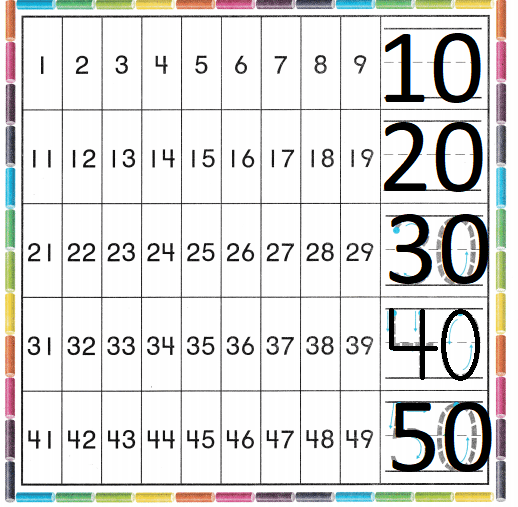Explanation:
The given pattern is count by tens
10 , 20 , 30 , 40 , 50 , 60 , 70 , 80 , 90 , 100

Directions
1. Write the numbers to complete the counting order to 20. Trace the numbers to complete the counting order to 50. Count by tens as you point to the numbers you wrote and traced.

Question 2.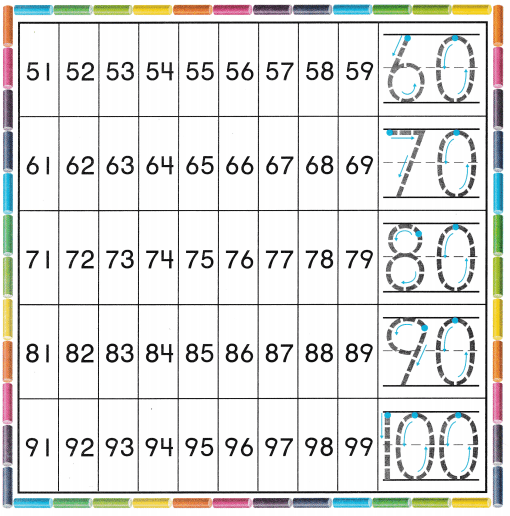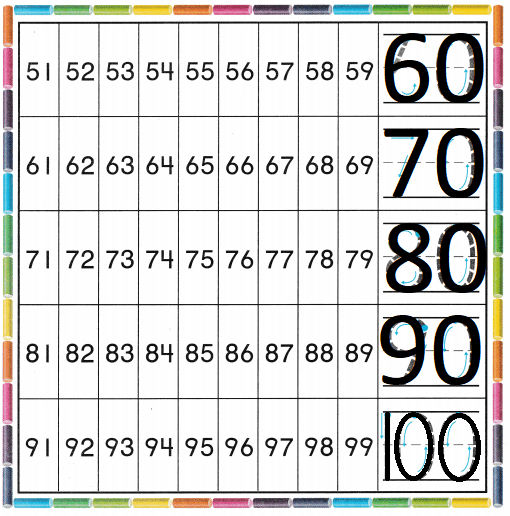Explanation:
The given pattern is count by tens
10 , 20 , 30 , 40 , 50 , 60 , 70 , 80 , 90 , 100

Directions
2. Trace the numbers to complete the counting order to 100. Count by tens as you point to the numbers you traced.

Home Activity

• Show your child a calendar. Use self-stick notes to cover random numbers. Ask your child to say the numbers that are covered. Then have him or her remove the self-stick notes to check.

Problem Solving

Question 3.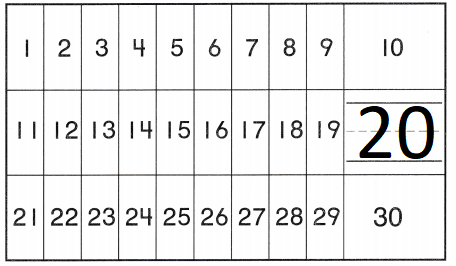Explanation:
The given pattern is count by tens
10 , 20 , 30 , 40 , 50 , 60 , 70 , 80 , 90 , 100
The missing number is 20

Question 4.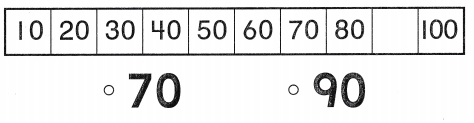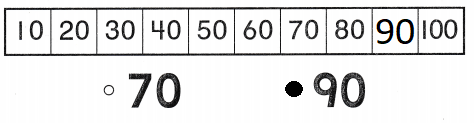Explanation:
The given pattern is count by tens
10 , 20 , 30 , 40 , 50 , 60 , 70 , 80 , 90 , 100
The missing number is 90

Directions
3. Listen to the problem. What is the last number Jenny says? Write the number. What is the lost number that Lindsay will say? Draw a line under the number. 4. Choose the correct answer. When you count by tens, what number comes after 80?

### Texas Go Math Kindergarten Lesson 16.3 Homework and Practice

Question 1.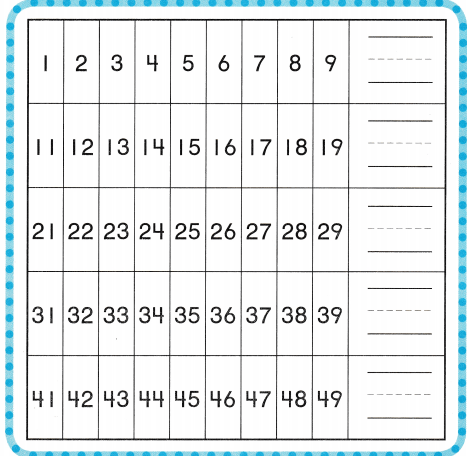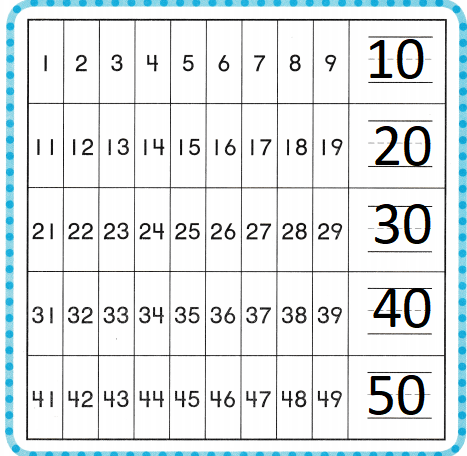Explanation:
The given pattern is count by tens
10 , 20 , 30 , 40 , 50 , 60 , 70 , 80 , 90 , 100

Directions
1. Write the numbers to complete the counting order to 50. Count by tens as you point to the numbers you wrote.

Texas Test Prep

Lesson Check

Question 2.Explanation:
The given pattern is count by tens
10 , 20 , 30 , 40 , 50 , 60 , 70 , 80 , 90 , 100
The missing number is 50

Question 3.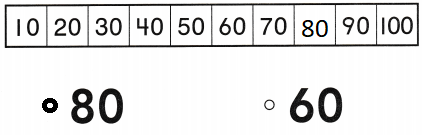Explanation:
The given pattern is count by tens
10 , 20 , 30 , 40 , 50 , 60 , 70 , 80 , 90 , 100
The missing number is 80.

Question 4.